# AC Bridges - 1 Notes | Study Electrical Engineering SSC JE (Technical) - Electrical Engineering (EE)

## Electrical Engineering (EE): AC Bridges - 1 Notes | Study Electrical Engineering SSC JE (Technical) - Electrical Engineering (EE)

The document AC Bridges - 1 Notes | Study Electrical Engineering SSC JE (Technical) - Electrical Engineering (EE) is a part of the Electrical Engineering (EE) Course Electrical Engineering SSC JE (Technical).
All you need of Electrical Engineering (EE) at this link: Electrical Engineering (EE)

What are AC Bridges?

The “AC Bridges” is a natural outgrowth of the DC bridge (wheatstone bridge) in its basic form consists of Four bridge arms a source of excitation and a null or balanced detector.

These bridge methods are very useful for the measurement of:

• Inductance (L)
• Capacitance (C)
• Frequency (f)
• Mutual inductance (M)
• Storage factor
• Loss factor, etc.

Types of Sources in AC Bridges

1. For Low frequency measurement the power line supply can serve as the source of excitation.
2. For High frequency measurement the electronic oscillator is used as excitation voltage.

Types of Detectors in AC Bridges

• It is used at frequencies of 250 Hz and over upto 3 to 4 KHz.
• Most sensitive detector for this ranges of frequency.

2. Vibration Galvanometer

• It can be used from 5 Hz to 1000 Hz but suitable mainly upto 200 Hz.
• They are extremely useful for power and low AF ranges.

• It can be used at 10 Hz to 100 KHz.

4. Cathode Ray Oscilloscope (CRO)

• It is used for higher frequency more than 5 KHz.

Note: For a DC Bridge , the “PMMC ” instrument acts as a detector.

AC Bridges through which Inductance (L) is Measured
1. Maxwell’s Inductance Bridge

This bridge measures an unknown inductance by comparison with a variable standard self inductance.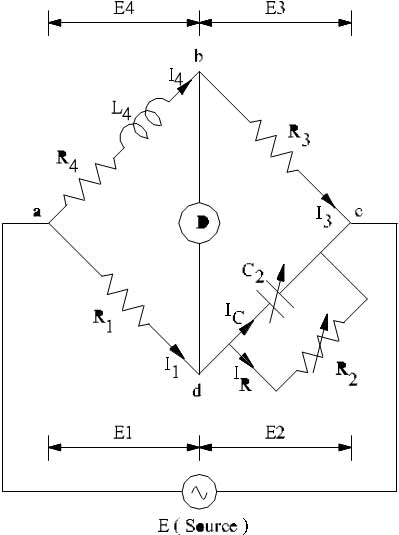Let R1 and L1 are unknown quantities.
L2 = Variable inductance of fixed resistance ‘r2
R2 = Variable resistance connected in series with “L2
R3 and R4 = Known non-inductive resistances.
At balance condition,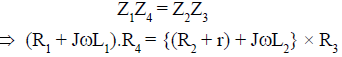equation real and imaginary part we get,

and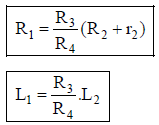2. Maxwell’s Inductance-Capacitance Bridge

This Bridge measures an unknown inductance in terms of a known capacitance.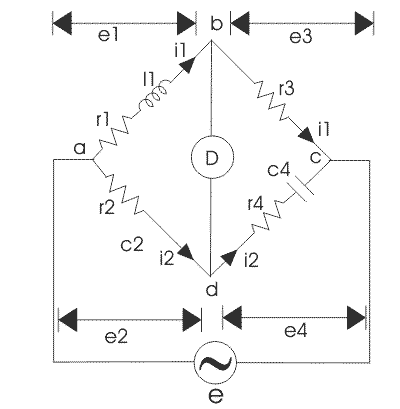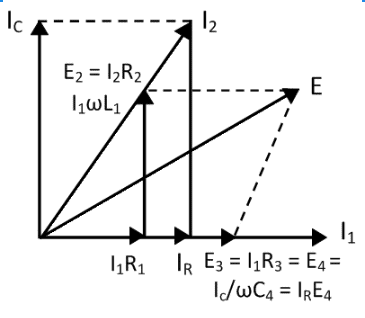Let R1 and Lare unknown quantity R2, R3 and Rare known non-inductive resistances and C4 = variable standard capacitor
At balance condition, Z1Z4 = Z2Z3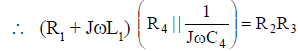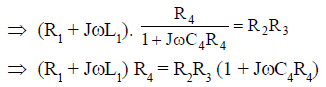Equating real part we get,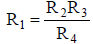and equating imaginary part we get,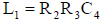Quality Factor: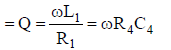• Circuit is simple.
• Obtained balance equations are free from the frequency term.
• Balance equations are independent if we choose R4 and C4 as variable elements.
• It is very useful for measurement of a wise range of inductances at power and audio frequencies.

• It requires variable standard capacitor which is very costly.
• The bridge is limited to measurement of low quality coils (1 < Q < 10) and it is also unsuitable for low value of Q (i.e. Q < 1) from this we conclude that a Maxwell bridge is used suitable only for medium Q coils.

3. HAY’s Bridge
• It is a modification of Maxwell’s bridge.
• This bridge uses a resistances in series with the standard capacitor as shown in figure below,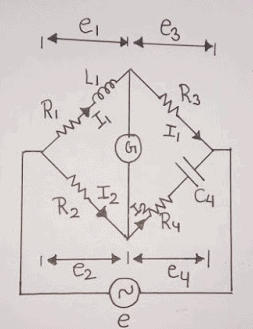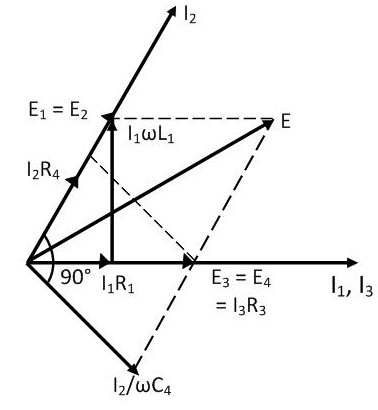Let,R1 and L1 are unknown quantity
R2, R3 and R4 are non-inductive resistances.
and C4 = standard capacitor
At balance condition,
Z1Z= Z2Z3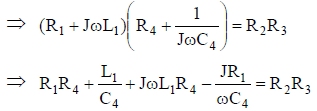Equating real and imaginary parts we get,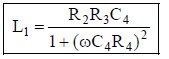and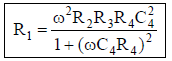Quality factor =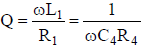i.e.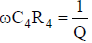Now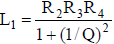and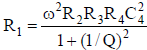• In this bridge, the expression for the unknown inductance and resistance involves the frequency term.
So, for the higher Q (i.e. Q > 10) 1 + (1/Q)2 ≈ 1 and then,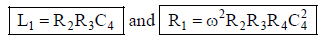• So, we can say this bridge is suitable for high Q-coils (i.e. Q > 10)

• It gives a simple expression for Q-factor.
• For high Q-coils it gives simple expression for unknown R1 and L1.

• It is not suitable for medium or low Q-coils.

4. Anderson’s Bridge

• It is a modification of the Maxwell ’s inductance-capacitance bridge.
• In this bridge method, the self inductance is measured in terms of standard capacitor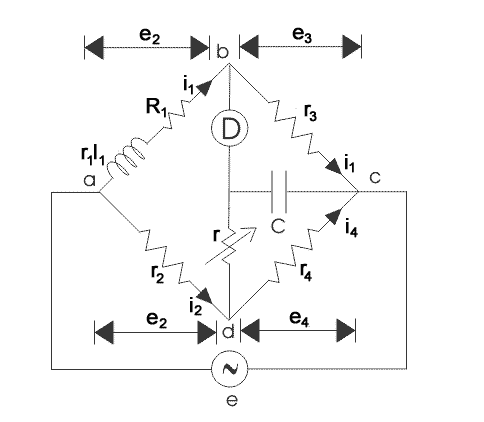At balanced condition, Vb = Ve

So, ID = 0
Also for the Delta network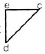we can convert this in star form

as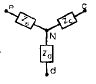So,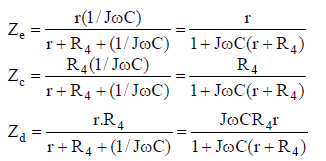For balance condition, Zab.Zc = ZaN.Zbc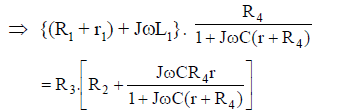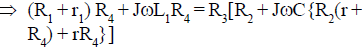Equating real part we get,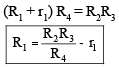Now equating imaginary part we get,
L1 R4 = R3C[R2 R4 + rR2 + rR4]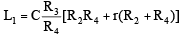Phasor diagram for Anderson’s bridge n/w: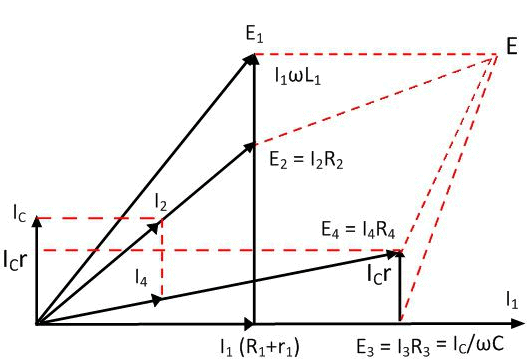Quality factor =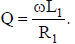• For Low Q-coil, L1 and C  So, it is suitable for Low Q-coils (i.e. Q < 1).
• It may be used for accurate estimation of capacitance in terms of inductance.
• It is relatively cheaper because here fixed capacitance is used.
• It is much easier to obtain the balance.

• It is more complex circuit.
• The balance equations are not simple and in fact are much more tedious.
• An additional junction point increases the difficulty of shielding the bridge network.

5. Owen’s Bridge

• This bridge may also be used for the measurement of inductance in terms of capacitance.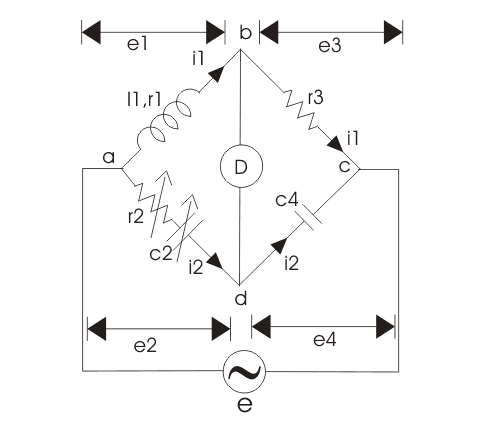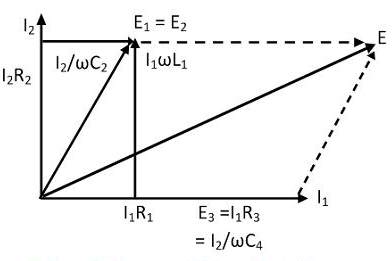Let R1 and L1 are the unknown quantity

R2 = Variable non-inductive resistance

R3 = Fixed non-inductive resistance

C2 = Variable standard capacitor

C= Fixed standard capacitor

At balance condition,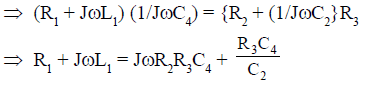Equating real and imaginary part we get,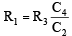and

L1 = R2 R3 C4
Quality factor  =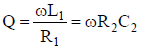• It has independent balance equations.
• The unknown quantities expressions are free from frequency term.
This can be used over a wide range of measurement of inductances.

• It requires a variable capacitor so it is very costly network.
• Its accuracy is about only 1%.

AC bridges through which capacitances are measured
1. De Sauty’s bridge
2. Schering bridge
1. De Sauty’s Bridge:
• It is the simplest method of comparing two capacitances.
• It may also be used for determining the dissipation factor (D).
Note: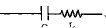then D = tan δ = ωCs rs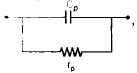then D = tan

δ = 1/ωCp rp

Considering ideal capacitors, the bridge circuit is,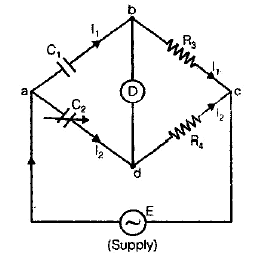Let C= unknown capacitor
C2 = a standard capacitor
R3 and R4 = non-inductive resistors
At balance condition:
Zab . Zcd = Zad . Zbc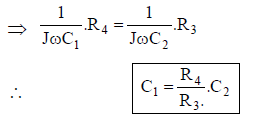The advantage of this bridge is its simplicity but from this we can not determine “Dissipation Factor (D)”, so some modification are needed in the above bridge.
Now we consider the lossy capacitor and bridge in becomes “Modified De-Sauty’s Bridge”.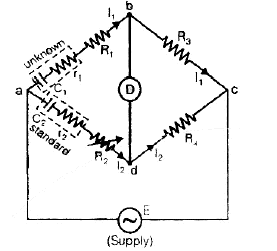• Given C2 so, D2 = ωC2r2 and we have to estimate C1 and hence D1 = ωC1r
At balance condition,
Zab . Zcd = Zbc . Zad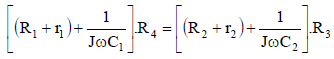Equating real part we get, (R1 + r1)R= (R2 + r2)R3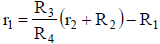Now equating imaginary part we have,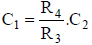and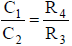Also,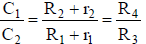• This bridge can not determine the accurate result for dissipation factor because we have,
D 2 – D1 = ω[C2r2 – C1r1]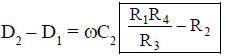Since (D2 – D1) is very-very small so its is difficult to determine “D1” accurately.

2. Schering Bridge

The Schering bridge use for measuring the capacitance of the capacitor, dissipation factor, properties of an insulator, capacitor bushing, insulating oil and other insulating materials. It is one of the most commonly used AC bridge. The Schering bridge works on the principle of balancing the load on its arm.

In this diagram: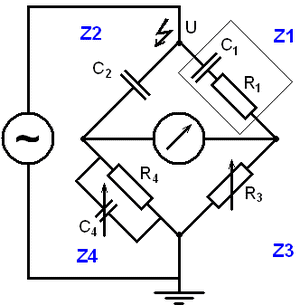• C1 = capacitor whose capacitance is to be determined
• R1 = a series resistance representing the loss in the capacitor C1
• C2 = a standard capacitor
• R3 = a variable non-inductive resistance
• C= a variable capacitor
• R4 = a non-inductive resistance in parallel with the variable capacitor C4

When the bridge is in the balanced condition, zero current passes through the detector, which shows that the potential across the detector is zero. At balance condition
Z1/Z2 = Z3/Z4
Z1Z4 = Z2Z3

Substituting the values of z1, z2, z3 and z4 in the above equation, we get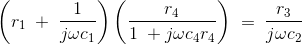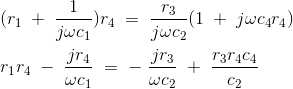Equating the real and imaginary parts and the separating we get,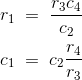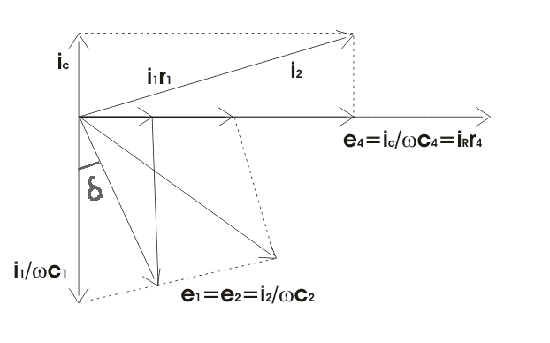Let us consider the phasor diagram of the above Schering bridge circuit and mark the voltage drops across ab,bc,cd and ad as e1, e3,e4 and e2 respectively. From the above Schering bridge phasor diagram, we can calculate the value of tanδ which is also called the dissipation factor.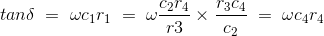The equation that we have derived above is quite simple and the dissipation factor can be calculated easily.

1. Balance equations are free from frequency.
2. The arrangement of the bridge is less costly as compared to the other bridges.

The document AC Bridges - 1 Notes | Study Electrical Engineering SSC JE (Technical) - Electrical Engineering (EE) is a part of the Electrical Engineering (EE) Course Electrical Engineering SSC JE (Technical).
All you need of Electrical Engineering (EE) at this link: Electrical Engineering (EE)Use Code STAYHOME200 and get INR 200 additional OFF

## Electrical Engineering SSC JE (Technical)

2 videos|65 docs|37 tests

Track your progress, build streaks, highlight & save important lessons and more!

,

,

,

,

,

,

,

,

,

,

,

,

,

,

,

,

,

,

,

,

,

;